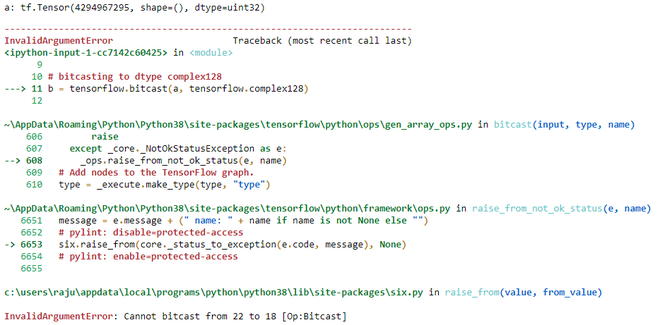GeeksforGeeks App
Open AppBrowser
Continue

# Python | tensorflow.bitcast() method

TensorFlow is open-source python library designed by Google to develop Machine Learning models  and deep learning  neural networks.

bitcast() is method in tensorflow library which is used to bitcast a tensor from one type to another type. It doesn’t copy the data.

```Syntax:
tf.bitcast(
input, type, name
)

Arguments:
1. input: It is the Tensor and the allowed type for this tensor are
bfloat16, half, float32, float64, int64, int32, uint8, uint16, uint32,
uint64, int8, int16, complex64, complex128, qint8, quint8, qint16, quint16, qint32.
2. type: It defines the dtype in which input need to be bitcasted.
3. name: It is an optional argument. It is used to give a name to operation.

Return: It returns a tensor of type type.```

Note: bitcast can’t be used to cast real dtype to complex dtype. It will raise InvalidArgumentError.

Example 1:

## Python3

 `# importing the library``import` `tensorflow` `# initializing the constant tensor of dtype uint32``a ``=` `tensorflow.constant(``0xffffffff``, dtype``=``tensorflow.uint32)` `# Checking the initialized tensor``print``(``'a:'``,a)` `# bitcasting to dtype uint8``b ``=` `tensorflow.bitcast(a, tensorflow.uint8)` `# Checking the bitcasted tensor``print``(``'b:'``,b)`

Output:

```a: tf.Tensor(4294967295, shape=(), dtype=uint32)
b: tf.Tensor([255 255 255 255], shape=(4,), dtype=uint8)```

Example 2:

This example tries to bitcast a real dtype to complex dtype

## Python3

 `# importing the library``import` `tensorflow` `# initializing the constant tensor of dtype uint32``a ``=` `tensorflow.constant(``0xffffffff``, dtype``=``tensorflow.uint32)` `# Checking the initialized tensor``print``(``'a:'``,a)` `# bitcasting to dtype complex128``b ``=` `tensorflow.bitcast(a, tensorflow.complex128)`

Output:My Personal Notes arrow_drop_up